Importance: Medium ✭✭
 Author(s): Macajova, Edita Skoviera, Martin
 Subject: Graph Theory » Basic Graph Theory » » Matchings
 Keywords: cubic nowhere-zero flow perfect matching
 Posted by: mdevos on: August 30th, 2007
Conjecture   Every bridgeless cubic graph has two perfect matchings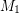,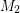so thatdoes not contain an odd edge-cut.

Let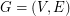be a bridgeless cubic graph. A binary cycle (henceforth called cycle) is a set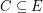so that every vertex ofhas even degree (equivalently, a cycle is any member of the binary cycle space). A postman join is a setso that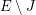is a cycle. Note that sinceis cubic, every perfect matching is a postman join. Next we state a well-known theorem of Jaeger in three equivalent forms.

Theorem  (Jaeger's 8-flow theorem)
\itemhas a nowhere-zero flow in the group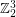. \itemhas three cycles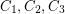so that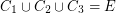. \itemhas three postman joins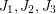so that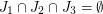.

The last of these statements is interesting, since The Berge Fulkerson Conjecture (if true) implies the following:

Conjecturehas three perfect matchingsso that.

So, we know thathas three postman joinswith empty intersection, and it is conjectured thatmay be chosen so that each is a perfect matching, but now we see two statements in between the theorem and the conjecture. Namely, is it true thatmay be chosen so that one is a perfect matching? or two? The first of these was solved recently.

Theorem  (Macajova, Skoviera)has two postman setsand one perfect matching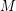so that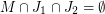The second of these asks for two perfect matchingsand one postman join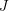so that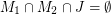. It is an easy exercise to show that a set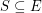contains a postman join if an only ifhas nonempty intersection with every odd edge-cut. Therefore, finding two perfect matchings and one postman join with empty common intersection is precisely equivalent to the conjecture at the start of this page - find two perfect matchings whose intersection contains no odd edge-cut.

## Bibliography

* Edita Macajova, Martin Skoviera, Fano colourings of cubic graphs and the Fulkerson conjecture. Theoret. Comput. Sci. 349 (2005), no. 1, 112--120. MathSciNet

* indicates original appearance(s) of problem.